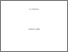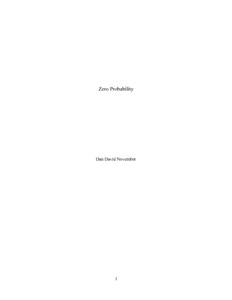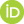# Zero Probability

November, Dan D (2019) Zero Probability. [Preprint]Preview
Text
Zero Probability - philsci-archive d3.pdf

## Abstract

In probability textbooks, it is widely claimed that zero probability does not mean impossibility. But what stands behind this claim? In this paper I offer an explanation for this claim based on Kolmogorov's formalism. As such, this explanation is relevant to all interpretations of Kolmogorov's probability theory. I start by clarifying that the claim refers only to nonempty events, since empty events are always considered impossible. Then I offer the following three reasons for the claim that nonempty events with zero probability are considered possible: The main reason is simply that they are nonempty, and so they are considered possible despite their zero probability. The second reason is that sometimes the zero probability is taken to be an approximation of some infinitesimal probability value. Such a value is strictly positive and as such does not imply impossibility in a strict sense. The third reason is that, according to some interpretations of probability, the same event can have different probabilities. They assume that an event with exactly zero probability (which does not approximate an infinitesimal value) can have strictly positive probabilities. This means that such an event can be possible, which implies that its zero probability does not mean impossibility.

 Export/Citation: EndNote | BibTeX | Dublin Core | ASCII/Text Citation (Chicago) | HTML Citation | OpenURL
 Social Networking:

Item Type: Preprint
Creators:
CreatorsEmailORCIDNovember, Dan Ddanny.november@mail.huji.ac.il
Subjects: Specific Sciences > Probability/Statistics
Depositing User: Mr. Dan D November
Date Deposited: 30 Jan 2019 19:34
Item ID: 15692
Subjects: Specific Sciences > Probability/Statistics
Date: 30 January 2019
URI: http://philsci-archive.pitt.edu/id/eprint/15692

### Monthly Views for the past 3 yearsView Item